# Troops

The route is long 147 km and the first-day first regiment went at an average speed of 12 km/h and journey back 21 km/h. The second day went second regiment the same route at an average speed of 22 km/h there and back.

Which regiment will take route longer?

Result

#### Solution: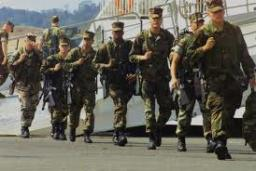Our examples were largely sent or created by pupils and students themselves. Therefore, we would be pleased if you could send us any errors you found, spelling mistakes, or rephasing the example. Thank you!

Please write to us with your comment on the math problem or ask something. Thank you for helping each other - students, teachers, parents, and problem authors.

Showing 1 comment:Math student
3qne=b=3wn8e b=Tips to related online calculators
Need help calculate sum, simplify or multiply fractions? Try our fraction calculator.
Check out our ratio calculator.
Do you have a linear equation or system of equations and looking for its solution? Or do you have quadratic equation?
Do you want to convert length units?
Do you want to convert velocity (speed) units?
Do you want to convert time units like minutes to seconds?

## Next similar math problems:

• Tourist routeHow long is the tourist route when tourists crossed four-sevenths of the way on foot, crossed the bus twice less than on foot and passed the last 14 kilometers by boat.
• Divide in ratioLine segment AB 12 cm long divide in a ratio of 5: 3. How long are the individual parts?
• The tourist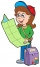The tourist walked a quarter of the way on the first day, a third of the rest on the second day, and 20 km on the last day. How many km did he walked in three days?
• Final exam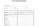At the final exam, the student answers from three areas, which are evaluated in a ratio of 1: 2: 2. What grade will John receive if he answered as follows: 3,1,2.How many times does the surface of a sphere decrease if we reduce its radius twice?
• Ratio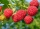Alena collected 7.8 kg of blueberries, 2.6 kg of blackberries, and 3.9 kg of cranberries. Express the ratio in the smallest natural numbers in this order.
• A farmer's heritage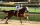A farmer died leaving his 17 horses to his 3 sons. When his sons opened up the Will it read: My eldest son should get 1/2 (half) of total horses; My middle son should be given 1/3rd (one-third) of the total horses; My youngest son should be given 1/9th (
• What time is it?What time is it, when there is 4 times less time left until midnight than the time that has elapsed since noon? What time is it, when one-fifth of the hours that have passed since midnight is equal to one-third of the hours that are missing by 12 o'clock?
• Double ratio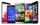The mobile phone was twice gradually discounted in the ratio of 3: 2 1 half: 5 quarters. How much did it originally cost if the price was CZK 4,200 after a double discount?
• Five combers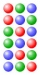Five combers harvest 12 rows of strawberries in 4 hours. How many rows of strawberries will two combers harvest in 10 hours?
• Age of familyThe age of father and son is in the ratio 10: 3. The age of the father and daughter is in ratio 5: 2. How old are a father and a son if the daughter is 20?
• Brass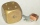Brass is an alloy of copper and zinc in a ratio of 3: 2. How many grams does a component that required 270 g of copper weigh?
• Half of halvesHalf of the square we cut off, then half of the rest, etc. Five cuts we made in this way. What part of the content of the original square is the content of the cut part?
• Interior anglesCalculate the interior angles of a triangle that are in the ratio 2: 3: 4.
• The angles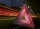The angles in the triangle are in the ratio 12: 15: 9. Find the angles.
• Cllub flag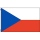The children had the club flag sewn on. The red color covered 2/20 of the total area of the flag, the blue area a fifth, the yellow 19% of the total area. What% is the green area occupied?
• The fourThe four pirates divided 65 coins to each other. They were sorted by age, the youngest receiving the least number of coins, each half more than the previous one. How many coins did the oldest pirate receive?